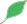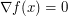## Numerical optimizationThis article is a stub.This means that it cannot be considered to contain or lead to any mathematically interesting information.

### Quick description

Numerical optimization is a large and important part of numerical analysis, with a great many practical applications.

We can focus on different types of problems, particularly, unconstrained and constrained (equality and inequality constrints). Constrained problems lead to the use of Lagrange multipliers. The objective function (the function to minimize or maximize) can be convex, smooth, or non-smooth (and convex or not as the case may be).

Non-convex problems are generally harder than convex ones, especially as there is often a problem of local minima (or local maxima).

### Prerequisites

Multivariate calculus.

### General discussion

Convex vs non-convex optimization.

Convex optimization is considered easier as finding a point satisfying(in the unconstrained case) or satisfying the Karush–Kuhn–Tucker conditions (in the general constrained case) is sufficient to show that the point is a global minimizer. For general non-convex optimization, this is not true.

Non-convex optimization in general requires some kind of global search; unless there is some special structure to the problem, derivative information can only determine the behaviour of a function nearby.

Algorithmic issues

Numerical optimization is about the application of computational methods, and so is inherently about (numerical) algorithms.